# Physics Test 7 - Ray And Wave Optics

## 25 Questions MCQ Test Mock Test Series for JEE Main & Advanced 2022 | Physics Test 7 - Ray And Wave Optics

Description
Attempt Physics Test 7 - Ray And Wave Optics | 25 questions in 60 minutes | Mock test for JEE preparation | Free important questions MCQ to study Mock Test Series for JEE Main & Advanced 2022 for JEE Exam | Download free PDF with solutions
QUESTION: 1

### The sun subtends an angle of half a degree at the pole of a concave mirror which has a radius of curvature of 15 metres. Then the size (disameter) of the image of the sun formed by the concave mirror is

Solution:
See , in this case ,
u = ∞ , Let x be the diameter ,
using mirror formula ,
1/u + 1/v = 1/f
v = f ,
let ∆ be the angle ,
∆ = x/f
x = ∆f ,
Multiply these two u will get diameter , or u can change your angle to radian.
QUESTION: 2

### A convex lens of focal length 24 cms is placed 12 cms in front of a convex mirror. It is found that when a pin is placed 36 cms in front of the lens, it coincides with its own inverted image formed by the lens and the mirror.Then the focal length of the mirror is

Solution: A convex lens of focal length 24 cm is placed 12 cm in front of a convex mirror. It is found that when a pin is placed 36 cm in front of the lens, it coincides with its own inverted image formed by the lens and the mirror. Then the focal length of the mirror is 30 cm.
QUESTION: 3

### A ray of light makes an angles of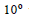with the horizontal and strikes a plane mirror which is inclinedat an angle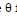to the horizontal.The anglefor which the reflected ray becomes vertical, is

Solution:
Draw a ray-diagram,
Angle between the reflected and incident ray = 80degree (Final ray is vertical and incidence is 10 wrt horizontal)
Angle of incidence = angle of reflected ray = 40degree
Angle of reflected ray with mirror = 90degree – 40degree = 50degree
So, angle with horizontal = 90degree – 50degree = 40degree
QUESTION: 4

The refractive index of a given piece of transparent quartz if greatest for

Solution: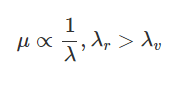CORRECT OPTION IS (B).
QUESTION: 5

If the critical angle for total internal reflection from a medium to vacuum is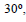the velocity of light in
the medium is

Solution:
We should know Snell's law
n1 sin I = n2 sin r
for critical angle the value of r becomes 90 degree
n1 / n2 = sin 90 / sin 30 = 2
also
n1/n2 = v2/v1
2 = 3 * 10^8 / velocity in medium
v= 1.5 * 10^8
QUESTION: 6
Two immiscible liquids are in a cylindrical vessel of depths of 8.4 cm and 7.8 cm, their refractive indices being 1.2 and 1.3 respectively. An object O at the bottom isseen from vertically
above the liquids. The upward displacement in cm in its apparant position is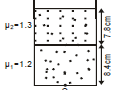Solution:
QUESTION: 7

X is a small angled prism of angle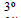made of a material of refractive index 1.5. A ray of light is made incident as shown in figure. M is a plane mirror.The angle of deviation for the ray reflected from the mirror. M with respect to incident ray is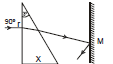Solution:
QUESTION: 8
The face AC of a prism ABC of refracting angle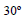is silvered. A ray is incident on face AB at an angle of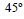as shown in figure. The refracted ray undergoes reflection on face AC and retraces its path. The refractive index of the prisms is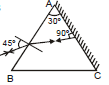Solution: Use triangle property180 - (90 + 30 ) = 60r = (90 - 60) = 30sin i/sin r = Refractive Index (R I)R I = sin 45/sin 30 = √2
QUESTION: 9
If half the body of a lens is covered with black paper the image produced by the lens will
Solution:
QUESTION: 10
The distance between the object and the screen is D (greater than 4 times the focal length of a convex lens). Real images of the object are obtained on the screen for two positions of the lens that are separated by distance d apart. The ratio of size of the images in the two positions of the lens are
Solution:
QUESTION: 11
An object 20 cm high produces using a lens a real image of 80cm.When an object is shifted by 5 cm a real image 40 cm high is produced.The power of the lens is
Solution:
QUESTION: 12
When the object is at a distance x1 and x2, a real and a virtual image of the same size are formed
respectively. The focal length of the convex lens is
Solution:
QUESTION: 13
An isotropic source of 2 candela  produces a light flux equal to
Solution:
QUESTION: 14
A lamp is hanging at a height 40 cm from the center of a table. If its height is increased by 10 cm, the illuminance on the table will decrease by
Solution:
QUESTION: 15
The intensity of light at a distance r from the axis of a long cylindrical source is proportional to
Solution:
QUESTION: 16
Rainbows are produced when sunlight
Solution:
QUESTION: 17
The magnifying power of telescope can be increased by
Solution:
QUESTION: 18
A movie projector projects an image of length 3.5 m of a 35 mm. movie film on a screen. Neglecting
absorption of light by the film and the air, the ratio of the illumination on the screen is
Solution:
QUESTION: 19
The focal length of a simple convex lens used as a magnifier is 10 cm. For the image to be formed at a distance of distinct vision (D = 25 cm), the object
must be placed away from the lens at a distance of
Solution: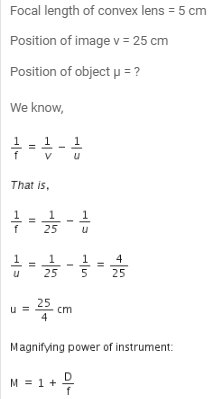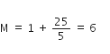QUESTION: 20
A person can see objects only at distance greater than 40 cm. He is advised to use lens of power
Solution: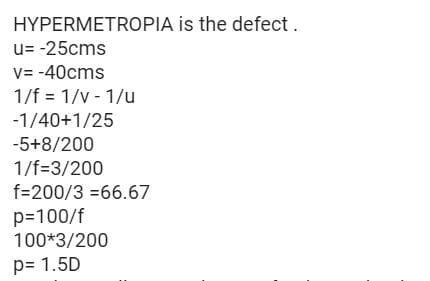*Answer can only contain numeric values
QUESTION: 21

A compound microscope has an eyepiece of focal length 10 cm and an objective of focal length 4 cm. Calculate the magnification, if an object is kept at a distance of 5 cm from the objective so that final image is formed at the least distance of distinct vision (20 cm) :-

Solution:
*Answer can only contain numeric values
QUESTION: 22

An object is placed on the axis of a convex lens. Its image is formed 80 cm from the object. The magnification is 3. The focal length of the lens (in cm) is:-

Solution:
*Answer can only contain numeric values
QUESTION: 23

A linear aperture whose width is 0.02 cm is placed immediately in front of a lens of focal length 60 cm. The aperture is illuminated normally by a parallel beam of wavelength 5 × 10–5 cm. The distance of the first dark band of the diffraction pattern from the centre of the screen is :-

Solution:

f = D = 60 cm
For first minima,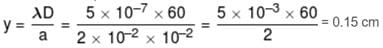*Answer can only contain numeric values
QUESTION: 24

Young's double slit experment is first performed in air and then in a medium other than air. It is found that 8th bright fringe in the medium lies where 5th dark fringe lies in air. The refractive index of the medium is nearly :-

Solution: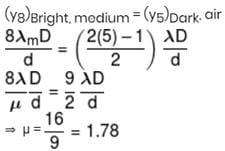*Answer can only contain numeric values
QUESTION: 25

Find the ratio of refractive index of transmitted medium with that of incident medium when the Brewster angle is 60 degree.

Solution: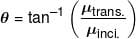Use Code STAYHOME200 and get INR 200 additional OFF Use Coupon Code